# Fejér sum

One of the arithmetic means of the partial sums of a Fourier series in the trigonometric system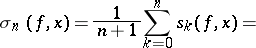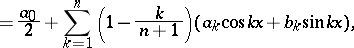where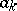andare the Fourier coefficients of the function.

Ifis continuous, thenconverges uniformly to;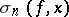converges toin the metric of.

Ifbelongs to the class of functions that satisfy a Lipschitz condition of order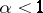, then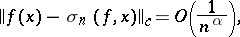that is, in this case the Fejér sum approximatesat the rate of the best approximating functions of the indicated class. But Fejér sums cannot provide a high rate of approximation: The estimate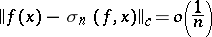is valid only for constant functions.

Fejér sums were introduced by L. Fejér .